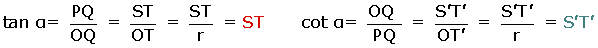Learn from home

The teachersThe unit circle has its center at (0, 0) and its radius is one.

In the unit circle, the coordinate axes delimit four quadrants that are numbered in an anti-clockwise direction.

By drawing a triangle on the unit circle, straight lines called line values can be drawn whose length represents the value of a trigonometric function.QOP and T'OS' are similar triangles.The sine is the ordinate, that is to say, the y-coordinate or the vertical value in a pair of coordinates.

The cosine is the abscissa, that is to say, the x-coordinate or the horizontal value in a pair of coordinates.

From this data, the sign of the trigonometric ratios in each quadrant are as follows:-1 ≤ sen α ≤ 1

-1 ≤ cos α ≤ 1Did you like the article?(1 votes, average: 5.00 out of 5)Loading...

Emma

I am passionate about travelling and currently live and work in Paris. I like to spend my time reading, gardening, running, learning languages and exploring new places.

Did you like
this resource?

Bravo!